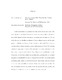## Two Goodness-of-Fit Tests for the Density Ratio Model2017
##### Authors
Yu, Luquan
Under consideration are goodness-of-fit tests for the \textit{density ratio model}. The model stipulates that the log-likelihood ratio of two unknown densities is of a known form which depends on finite dimensional parameters and a tilt function. We can derive the empirical distribution estimator $\tilde{G}$ from a reference sample, and the semiparametric distribution estimator $\hat{G}$ under the density ratio model. Furthermore we can derive kernel density estimators $\tilde{g}$ and $\hat{g}$ corresponding to $\tilde{G}$ and $\hat{G}$ by choosing a bandwidth parameter. Goodness-of-fit test statistics can be constructed via the discrepancy between $\tilde{g}$ and $\hat{g}$ using Hellinger distance and a modification thereof. We propose two new test statistics by modifying the goodness-of-fit test statistics suggested by Bondell (2007) and by Cheng and Chu (2004). Asymptotic results and limiting distributions are derived for both new test statistics, and the selections of the kernel and bandwidth are discussed. Monte-Carlo simulations show that the new test statistics improve the accuracy of the the goodness-of-fit test and that the limiting distributions of the new test statistics are more symmetric.